International Journal of Systems Science and Applied Mathematics
Volume 1, Issue 4, November 2016, Pages: 58-62

An Algorithm to Solve Fuzzy Trapezoidal Transshipment Problem

P. Gayathri1, K. R. Subramanian2

1Department of Mathematics, A.V.C.College (Autonomous), Mannampandal, Mayiladuthurai

2Department of Computer Applications, Srimati Indira Gandhi College, Trichy, Tamilnadu, India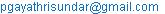(P. Gayathri)

P. Gayathri, K. R. Subramanian. An Algorithm to Solve Fuzzy Trapezoidal Transshipment Problem. International Journal of Systems Science and Applied Mathematics. Vol. 1, No. 4, 2016, pp. 58-62. doi: 10.11648/j.ijssam.20160104.14

Received: September 15, 2016; Accepted: October 10, 2016; Published: November 9, 2016

Abstract: The fuzzy transportation problem in which available commodity frequently moves from one source to another source or destination before reaching its actual destination is called a fuzzy transshipment problem. In this paper, a new method is proposed to find the fuzzy optimal solution of fuzzy transportation problems with the following transshipment: From a source to any another source, from a destination to another destination, and from a destination to any source. In the proposed method all the parameters are represented by trapezoidal fuzzy numbers. To illustrate the proposed method a fuzzy transportation problem with transshipment is solved. The proposed method is easy to understand and to apply for finding the fuzzy optimal solution of fuzzy transportation problems with transshipment occurring in real life situations.

Keywords: Transportation Problem, Fuzzy Transshipment Problem, Trapezoidal Fuzzy Numbers

Contents

1. Introduction

In a fuzzy transportation problem shipment of article of trade takes place between sources and destinations. But as an alternative of direct shipments to destinations, the commodity can be transported to a meticulous destination through one or more intermediated or fuzzy transshipment points. Each of these points in turn supply to other points. Thus, when the shipments pass from destination to destination and from source to source, fuzzy transshipments exists here. Such a problem cannot be solved as such by the usual fuzzy transportation algorithm, but slight alteration is required before applying to the fuzzy transshipment problem. Transshipment usually takes place in transport hubs. Much international transshipment also takes place in selected customs areas, thus avoiding the need for customs checks or duties, otherwise a major difficulty for efficient transport. For example, stockiest reserves the goods viz. medicines, food grains and other items at warehouses for emergencies. On the other hand, when there is an extra demand in the market due to high storage cost at some sources or during festive and marriage seasons or during fire and military services, the total flow needs to be improved compelling some of the factories to increase their productions in order to meet this extra demand. In literature, Transshipment Problem was first introduced by Orden . He gave an extension of the original transportation problem to include the possibility of transshipment i.e., any shipping or receiving point is also permitted to act as an intermediate point. The Transshipment technique is used to find the shortest route from one point in a network to another. The problem of determining simultaneously the flows of primary products through processors to the market as final products has been formulated alternatively as a transshipment model by King and Logan . An extension of this problem to a multiregional, multiproduct and multi-plant problem formulated in the general linear programming model has been proposed by Judge et al. . Afterwards, various alternatives formulations of the transshipment problem within the framework of the transportation model that permits solution of problems of the type discussed by King and Logan without the need for subtraction of artificial variables were discussed by Hurt and Tramel . Brigden  considered the transportation problem (TP) with mixed constraints. He solved this problem by considering a related standard transportation problem having two additional supply points and two additional destinations. Klingman and Russel  introduced a specialized method for solving a transportation problem with several additional linear constraints. In 1985, Garg and Prakash  studied time minimizing transshipment problem. After that Gupta et al. [8, 9] have also worked on linear and non-linear transportation problems. In the recent years, indefinite Quadratic Transportation Problem has also been studied [10, 12]. Dahiya and Verma  studied capacitated transportation problem with bounds on the rim conditions. Khurana et al. [13, 14] studied three dimensional time minimizing transshipment problem. In literature, much effort has been concentrated on transportation problems as well as transshipment problems with equality constraints. We have solved the problems for balanced as well unbalanced cases and have discussed the various situations emerging out of unbalanced transshipment problems. The algorithms and transformation are easy to understand and to apply. The solution method can serve as an effective tool to the managers having production allocation problems.

2. Preliminaries

In this section some basic definitions and arithmetic operations are reviewed.

2.1. Basic Definitions

In this section, some basic definitions are reviewed. 

1)  A fuzzy set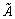is defined by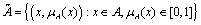.

In the pair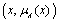, the first element x belong to the classical set A, the second element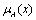belong to the interval [0, 1], called Membership function.

2)  A trapezoidal fuzzy number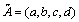is said to be non-negative trapezoidal fuzzy number if and only if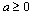.

3)  A trapezoidal fuzzy numberis said to be zero, if and only if a = 0, b = 0, c = 0, d = 0.

4)  Two trapezoidal fuzzy numbers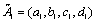and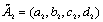are said to be equal i.e.,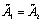if and only if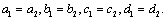5)  A ranking function is a function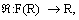where F(R) is a set of fuzzy numbers defined on set of real numbers, which maps each fuzzy number into the real line.

Letbe a trapezoidal fuzzy number then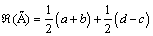2.2. Arithmetic Operations

Letandbe two trapezoidal fuzzy numbers then

(i)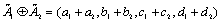(ii)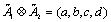where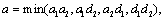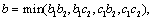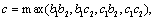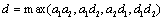3. Formulation of the Fuzzy Transshipment Problem

The fuzzy transportation problem assumes that direct routes exist from each source to each destination. However, there are situations in which units may be shipped from one source to another or to other destinations before reaching their destinations. This is called a fuzzy transshipment problem. The purpose of transshipment, the distinction between a source and destination is dropped so that a transportation problem with m sources and n destinations gives rise to a transshipment problem with m + n sources and m + n destinations. The basic feasible solution to such a problem will involve [(m + n) + (m + n) -1] or 2m + 2n -1 basic variables and if we omit the variables appearing in the (m + n) diagonal cells, we are left with m + n – 1 basic variables. Thus the fuzzy transshipment problem may be written as:

Maximize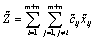Subject to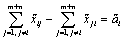, i = 1, 2, 3, …….., m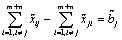, j= m + 1, m + 2, m + 3, …, m + n

where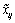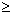0, I, j = 1, 2, 3, ……., m + n, j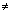i

where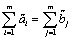then the problem is balanced otherwise unbalanced.

The above formulation is a fuzzy transshipment model, the transshipment model is reduced to transportation model:

MinimizeSubject to, i =1, 2, 3, …….., m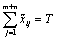, i = m + 1to m + n,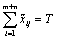, j =1 to m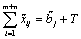, j= m + 1 to m + n

where0, i, j = 1 to m + n, ji

The above mathematical model represents a standard balanced transportation problem with (m + n) origins and (m + n) destinations. T can be interpreted as a buffer stock at each origin and destination. Since we assume that any amount o goods can be transshipped at each point, T should be large enough to take care of all transshipments. It is clear that the volume of goods transshipped at any point cannot exceed the amount produced or received and hence we take4. Proposed Algorithm

Step 1: Balance the given transshipment problem if either (total supply > total demand) or (total supply < total demand). Now the transshipment table in fuzzy environment looks like fuzzy transportation table.

Step 2: In this fuzzy transportation table all the cost, supply and demand are in fuzzy. Defuzzify all the cost elements in the balanced transshipment table.

Step 3: To apply the proposed algorithm, subtract the difference of largest and smallest cost elements of the above and below main diagonal elements from all the elements of the cost matrix in the transportation table.

Step 4: Arrange all the costs of transportation table in ascending order. (If the costs are repeated, consider it once). Assign numbers 1, 2, 3, ……to these arranged costs and form a new Transportation table with their corresponding numbers for the costs.

Step 5: Since the supply & demand are in fuzzy, for ranking of trapezoidal fuzzy numbers use the ranking formula mentioned in preliminaries. Find the total values assigned in the place of cost elements row-wise as well as column-wise.

Step 6: Identify a row (or column) for this max total and allocate the minimum trapezoidal Fuzzy number corresponding to supply (or demand). Allocate the min. fuzzy number of supply (or demand) corresponding to the min. cost of the identified row (or column)

Step 7: Subtract the allocated fuzzy number from the corresponding supply and demand values. Delete a row (or column) with zero supply (or demand). Continue this process until all rows and columns are satisfied.

Step 8: Compute the total fuzzy transportation cost for the feasible cost for the feasible allocation using the original balanced fuzzy transshipment cost matrix.

5. Numerical Example

Consider the following transshipment problem containing two sources and three destinations having demand and availability represented as tables values given below.

Table 1. Fuzzy Transshipment problem (Contains 4 tables as given below).

 S1 S2 S1 (0,0,0,0) (0.5,1,1,2) S2 (0.5,1,1,2) (0,0,0,0)
 D1 D2 AVAILABILITY S1 (1,4,1,3) (2,5,1,3) (3,6,2,4) S2 (0.2,2.8,5,2.5) (2,5,1,3) (13,28,8,26) DEMAND (12.3,21.2,6.5,19.5) (3.7,12.8,3.5,10.5)
 D1 D2 D1 (0,0,0,0) (0.5,1,1,2) D2 (0.5,1,1,2) (0,0,0,0)
 S1 S2 D1 (1,4,1,3) (0.2,2.8,5,2.5) D2 (2,5,1,3) (2,5,1,3) (16,34,10,30) (16,34,10,30)

Step1: The Unbalanced Transportation Table is given by

Table 2. Fuzzy Transportation problem.

 S1 S2 D1 D2 AVAILABILITY S1 (0,0,0,0) (0.5,1,1,2) (1,4,1,3) (2,5,1,3) (3,6,2,4) S2 (0.5,1,1,2) (0,0,0,0) (0.2,2.8,5,2.5) (2,5,1,3) (13,28,8,26) D1 (1,4,1,3) (0.2,2.8,5,2.5) (0,0,0,0) (0.5,1,1,2) - D2 (2,5,1,3) (2,5,1,3) (0.5,1,1,2) (0,0,0,0) - DEMAND - - (12.3,21.2,6.5,19.5) (3.7,12.8,3.5,10.5)

Step2: The Balanced Transportation Table is formed

Table 3. Fuzzy Balanced Transportation problem.

 S1 S2 D1 D2 AVAILABILITY S1 (0,0,0,0) (0.5,1,1,2) (1,4,1,3) (2,5,1,3) (19,40,12,34) S2 (0.5,1,1,2) (0,0,0,0) (0.2,2.8,5,2.5) (2,5,1,3) (29,62,18,56) D1 (1,4,1,3) (0.2,2.8,5,2.5) (0,0,0,0) (0.5,1,1,2) (16,34,10,30) D2 (2,5,1,3) (2,5,1,3) (0.5,1,1,2) (0,0,0,0) (16,34,10,30) DEMAND (16,34,10,30) (16,34,10,30) (28.3,55.2,16.5,49.5) (19.7,46.8,13.5,40.5)

Step 3: The Defuzzified Balanced Transportation Table is calculated by subtracting all the cost values from the difference of 4.5 and 0.25

Table 4. Defuzzified Transportation problem.

 S1 S2 D1 D2 AVAILABILITY S1 0 1.25 3.5 4.5 (19,40,12,34) S2 1.25 0 0.25 4.5 (29,62,18,56) D1 3.5 0.25 0 1.25 (16,34,10,30) D2 4.5 4.5 1.25 0 (16,34,10,30) DEMAND (16,34,10,30) (16,34,10,30) (28.3,55.2,16.5,49.5) (19.7,46.8,13.5,40.5)

Step 4: The revised Balanced Transportation Table is calculated by subtracting all the cost from the difference of 4.5 and 0.25 and assigning values 1,2,3… according to the values

Table 5. Revised Transportation problem.

 S1 S2 D1 D2 AVAILABILITY S1 1 3 4 5 (19,40,12,34) S2 3 1 2 5 (29,62,18,56) D1 4 2 1 3 (16,34,10,30) D2 5 5 3 1 (16,34,10,30) DEMAND (16,34,10,30) (16,34,10,30) (28.3,55.2,16.5,49.5) (19.7,46.8,13.5,40.5)

Step 5: The solved Transportation table is given by

Table 6. Solved Transportation problem.

 S1 S2 D1 D2 AVAILABILITY S1 1 3 4 5 (19,40,12,34) (16,34,10,30) (3,6,2,4) (3,6,2,4) S2 3 1 2 5 (29,62,18,56) (13,28,8,26) (16,34,10,30) (13,28,8,26) D1 4 2 1 3 (16,34,10,30) (12.3,21.2,6.5,19.5) (3.7,12.8,3.5,10.5) (12.3,21.2,6.5,19.5) D2 5 5 3 1 (16,34,10,30) (16,34,10,30) DEMAND (16,34,10,30) (16,34,10,30) (28.3,55.2,16.5,49.5) (19.7,46.8,13.5,40.5) (13,28,8,4) (16,34,10,30) (3.7,12.8,3.5,10.5)

Number of fuzzy units transported from origin to destinations S1 to S1 is (16,34,10,30), S1 to S2 is (3,6,2,4), S2 to S2 is (13,28,8,26), S2 to D1 is (16,34,10,30), D1 to D1 is (12.3,21.2,6.5,19.5), D1 to D2 is (3.7,12.8,3.5,10.5), D2 to D2 is (16,34,10,30).

The optimum fuzzy transshipment cost is (0.5,1,1,2)*(3,6,2,4)+(0.2,2.8,5,2.5)*(16,34,10,30)+(0.5,1,1,2)* (3.7,12.8,3.5,10.5) = 30.3125. For the above transshipment problem, the fuzzy transshipment cost by Linear Programming Problem method is 56.0375. Hence the proposed algorithm gives more optimum result than the result given by LPP method.

6. Conclusion

In this paper, an algorithm is developed to solve the fuzzy transshipment problem by using trapezoidal fuzzy number to get an optimum solution. The algorithm is easy to understand and less time consumable. Numerical example is solved to illustrate the theory. In today’s highly competitive market, the pressure on organizations to find better ways to create and deliver value to customers becomes stronger. How and when to send the products to the customers in the quantities, they want in a cost-effective manner, become more challenging. The optimum solution of Transshipment models provides a powerful framework to meet this challenge.

References

1. Orden A (1956) Transshipment problem. Manag Sci 2(3):276285.
2. King GA, Logan SH (1964) Optimum location, number, and size of processing plants with raw product and final product shipments. J Farm Econ 46:94108.
3. Judge GG, Havlicek J, Rizek RL (1965) An interregional model: its formulation and application to thelivestock industry. Agric Econ Rev 17:19.
4. Hurt VG, Tramel TE (1965) Alternative formulations of the transshipment problem. J Farm Econ47(3):763773.
5. Brigden MEV (1974) A variant of transportation problem in which the constraints are of mixed type.Oper Res Quaterly 25(3):437445.
6. Klingmana D, Russel R (1975) Solving constrained transportation problems. Oper Res 23(1):91105.
7. Garg R, Prakash S (1985) Time minimizing transshipment problem. Indian J Pure Appl Math 16(5):449460.
8. Gupta A, Khanna S, Puri MC (1992) Paradoxical situations in transportation problems. Cah CentEtudesde Rech Operationnell 34:3749.
9. Gupta A, Khanna S, Puri MC (1993) A paradox in linear fractional transportation problems with mixedconstraints. Optimization 27:375387.
10. Arora SR, Khurana A (2004) Three dimensional fixed charge bicriterion indefinite quadratictransportation problem. Yugoslavia J Oper Res 14(1):8397.
11. Dahiya K, Verma V (2007) Capacitated transportation problem with bounds on the rim conditions. Eur JOper Res 178:718737.
12. Khurana A, Thirwani D, Arora SR (2009) An algorithm for solving fixed charge bi-criterion indefinitequadratic transportation problem with restricted flow. Int J Optim Theory Methods Appl 1(4):367380.
13. Khurana A, Arora SR (2011) Fixed charge bi-criterion indefinite quadratic transportation problem withenhanced flow. Rev Investig Operacional 32:133145.
14. Khurana A, Verma T, Arora SR (2012) An algorithmfor solving time minimizing transshipmentproblem. Int J Manag Sci Eng Manag 7(3):192199.
15. Dubois, D., Prade, H.: Fuzzy Sets and Systems: Theory and Applications. Academic, New York (1980).

 Contents 1. 2. 2.1. 2.2. 3. 4. 5. 6.
Article ToolsAbstractPDF(184K)